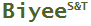Tweet

# Binomial Confidence Interval Calculator

## FREE on-line and off-line tools

(last updated on 2017-09-06)

This tool calculates a set of confidence intervals for the entered binomial trial result - the number of trials and the number of successes.

Researchers often want to know, after obtaining study results, how the same data can be interpreted differently with different confidence levels, and hence different confidence intervals.  Sometimes, they also wish to know, before a study, what are the possible conclusions based on different confidence levels assuming certain outcome.  This calculator can help them in this regard.

One-sided confidence intervals are particularly useful for reliability studies where only the lower bound of the average reliability is concerned.

Note:

• The confidence intervals are calculated using the exact method.  They are also called Clopper-Pearson intervals.  They are the most conservative type because they guarantee the coverage (i.e. the confidence level) for any population proportion.  The true coverage may be above the the confidence interval.
• The maximum of allowed N is 1000.
• It may take a moment to complete the calculation for a large N.

### Sorry, your browser does not support on-line Silverlight app.

Related articles: